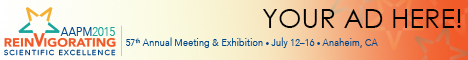# Program Information

## Adaptive-Beamlet Based Finite Size Pencil Beam (AB-FSPB) Dose Calculation Algorithm for Independent Verification of IMRT and VMATC Park

## Presentations

TH-E-BRE-11 Thursday 1:00PM - 2:50PM Room: Ballroom E

Purpose:In current IMRT and VMAT settings, the use of sophisticated dose calculation procedure is inevitable in order to account complex treatment field created by MLCs. As a consequence, independent volumetric dose verification procedure is time consuming which affect the efficiency of clinical workflow. In this study, the authors present an efficient Pencil Beam based dose calculation algorithm that minimizes the computational procedure while preserving the accuracy.

Methods:The computational time of Finite Size Pencil Beam (FSPB) algorithm is proportional to the number of infinitesimal identical beamlets that constitute the arbitrary field shape. In AB-FSPB, the dose distribution from each beamlet is mathematically modelled such that the sizes of beamlets to represent arbitrary field shape are no longer needed to be infinitesimal nor identical. In consequence, it is possible to represent arbitrary field shape with combinations of different sized and minimal number of beamlets.

Results:On comparing FSPB with AB-FSPB, the complexity of the algorithm has been reduced significantly. For 25 by 25 cm2 squared shaped field, 1 beamlet of 25 by 25 cm2 was sufficient to calculate dose in AB-FSPB, whereas in conventional FSPB, minimum 2500 beamlets of 0.5 by 0.5 cm2 size were needed to calculate dose that was comparable to the result computed from Treatment Planning System (TPS). The algorithm was also found to be GPU compatible to maximize its computational speed. On calculating 3D dose of IMRT (~30 control points) and VMAT plan (~90 control points) with grid size 2.0 mm (200 by 200 by 200), the dose could be computed within 3~5 and10~15 seconds.

Conclusion:Authors have developed an efficient Pencil Beam type dose calculation algorithm called AB-FSPB. The fast computation nature along with GPU compatibility has shown performance better than conventional FSPB. This completely enables the implantation of AB-FSPB in the clinical environment for independent volumetric dose verification.

Contact Email: